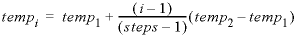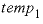| Next || Previous || Top |

## REACTION_TEMPERATURE

This keyword data block is used to define temperature during batch-reaction steps. This data block can also be used to specify the temperature in a cell or range of cells during advective-transport calculations ( ADVECTION ) and the initial temperature for a cell or range of cells in advective-dispersive transport calculations (TRANSPORT).

###### Example data block 1
`Line 0: REACTION_TEMPERATURE 1 Three explicit reaction temperatures.`
`Line 1:     15.0     25.0     35.0`
###### Explanation 1

Line 0: REACTION_TEMPERATURE [ number ] [ description ]

REACTION_TEMPERATURE is the keyword for the data block.

number --Positive number or a range of numbers to designate this temperature definition. A range of numbers may be given in the form m-n , where m and n are positive integers, m is less than n , and the two numbers are separated by a hyphen without intervening spaces. Default is 1.

description --Optional comment that describes the temperature data.

Line 1: list of temperatures

list of temperatures --A list of temperatures (°C) that will be applied to batch-reaction calculations. More lines may be used to supply additional temperatures. One batch-reaction calculation will be performed for each listed temperature.

###### Example data block 2
`Line 0: REACTION_TEMPERATURE 1 Three implicit reaction temperatures.`
`Line 1:      15.0     35.0 in 3 steps`
###### Explanation 2

Line 0: REACTION_TEMPERATURE [ number ] [ description ]

Same as Example data block 1.

Line 1: temp 1 , temp 2 , in steps

temp 1 --Temperature of first reaction step, °C.

temp 2 --Temperature of final reaction step, °C.

in steps --“ in ” indicates that the temperature will be calculated for each of steps number of steps. The temperature at each step, i , will be calculated by the formula; if steps = 1, then the temperature of the batch reaction will be. Example data block 2 performs exactly the same calculations as Example data block 1. If more batch-reaction steps are defined by KINETICS, REACTION, or REACTION_PRESSURE input, the temperature of the additional steps will be temp 2 .

###### Notes

If more batch-reaction steps are defined in KINETICS, REACTION, or REACTION_PRESSURE than temperature steps in REACTION_TEMPERATURE , then the final temperature will be used for all of the additional batch-reaction steps. INCREMENTAL_REACTIONS keyword has no effect on the REACTION_TEMPERATURE data block. The default temperature of a reaction step is equal to the temperature of the initial solution or the mixing-fraction-averaged temperature of a mixture. REACTION_TEMPERATURE input can be used even if there is no REACTION input. The method of calculation of temperature steps using “ in ” is slightly different than that for reaction steps. If n temperature steps are defined with “ in n ” in a REACTION_TEMPERATURE data block, then the temperature of the first reaction step is equal to temp 1 ; temperatures in the remaining steps change in n-1 equal increments. In contrast, if n reaction steps are defined with “ in n ” in a REACTION data block, then the reaction is added in n equal increments.

In an advective-transport calculation ( ADVECTION ), if REACTION_TEMPERATURE n is defined (or a range is defined n - m ), and n is less than or equal to the number of cells in the simulation, then the first temperature in the data block of REACTION_TEMPERATURE n is used as the temperature in cell n (or cells n - m ) for all shifts in the advective-transport calculation. In advective-dispersive transport simulations (TRANSPORT), the initial equilibration also occurs at the first temperature of REACTION_TEMPERATURE n in cell n . However, depending on the setting of temperature retardation factor ( -thermal_diffusion in the TRANSPORT data block), an exchange of heat may take place that will cause the temperature of the cell to change as transport progresses.

###### Example problems

The keyword REACTION_TEMPERATURE is used in example problems 2 and 22.

###### Related keywords

| Next || Previous || Top |